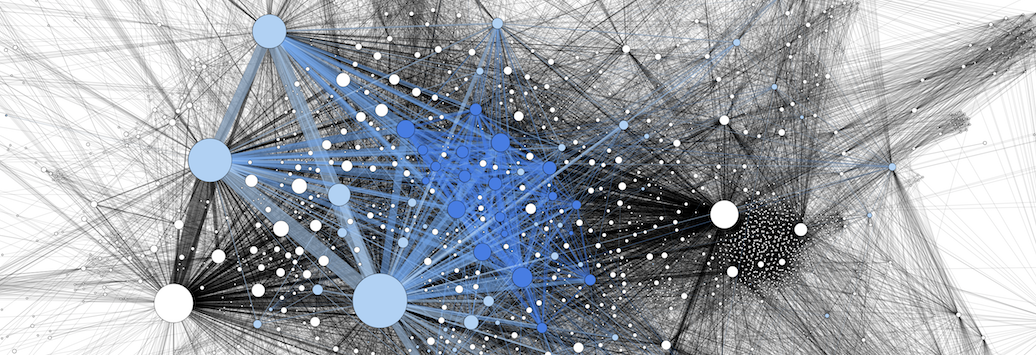# what is data science?Data science is the multidisciplinary field that uses scientific methods, processes, algorithms, and systems to obtain insights and information from structured and unstructured digital data. This data is usually very difficult to interpret by humans. Machines allow us to interpret, process, and alter this data very effectively.

Data science combines statistics, data analysis, machine learning, and their related methods in order to understand and analyze actual phenomena.

The digitization of data was not the only factor in data science’s emergence. Data sets had to become very large, while accessing and manipulating these data sets had to become very fast.

## Data Analysis

Due to the enormous scale of modern big data, finding meaning requires tools such as SQL, Python, or R. SQL, standing for “structured query language” is the language for accessing and manipulating databases. Python is a general purpose language that emphasizes code readability. R is a language and environment for statistical computing and graphics. These allow data analysts to aggregate and manipulate data to the point where they can present meaningful conclusions.

## Experimentation

Knowing how to run an effective experiment, keeping the data clean, and analyzing it appropriately is what makes up the life of a data scientist. Ineffective experimentation leads to biases, which leads to false conclusions.

## Machine Learning

Data scientists define machine learning (ML) as the scientific study of algorithms and statistical models that machines use to effectively perform a specific task without using explicit instructions, but instead relying on patterns and inference. It is seen as a subset of artificial intelligence. Machine learning algorithms build a mathematical model of sample data, known as “training data”, in order to make predictions or decisions without being explicitly programmed to perform that task.

Machine learning tasks can be classified into several broad categories: supervised learning, unsupervised learning, and reinforcement learning.

## Supervised Learning

Supervised or semi-supervised learning algorithms build a mathematical model of a set of data that contains both the inputs and the desired outputs. The data is known as “training data”, and consists of a set of training examples. Each training example has one or more inputs and a desired output, also known as a “supervisory signal”. For semi-supervised learning algorithms, some of the training examples do not include a desired output. In the mathematical model, each training example is represented by an array or vector, and the training data by a matrix.

Iterative optimizations of an “objective function” allow supervised learning algorithms to learn a function that, when optimal, can be used to correctly determine the output for inputs that were not a part of the training data. This optimized algorithm is said to have learned to perform that task.

Supervised learning algorithms include classification and regression. Classification algorithms are used when the outputs are restricted to a limited set of values and regression algorithms are used when the outputs may have any numerical value within a range.

## Unsupervised Learning

Unsupervised learning algorithms take a set of data that contains only inputs, and find structure in the data, like grouping or clustering of data points. These algorithms therefore learn from test data that has not been labeled, classified, or categorized. Instead of responding to feedback, unsupervised learning algorithms identify commonalities in the data and react based on the presence or absence of such commonalities in each new piece of data.

While supervised and unsupervised learning algorithms have different objectives, in real world problems they often take place simultaneously.

## Reinforcement Learning

Reinforcement learning (RL) is concerned with how software agents (or bots) ought to take actions under certain conditions, so as to maximize some notion of cumulative reward. In machine learning, the environment is typically represented as a Markov Decision Process (MDP). RL algorithms do not assume knowledge of an exact mathematical model of the MDP, and are used when exact models are infeasible.